International
Tables for
Crystallography
Volume B
Reciprocal space
Edited by U. Shmueli

International Tables for Crystallography (2010). Vol. B, ch. 1.5, p. 175   | 1 | 2 |

Section 1.5.1. List of abbreviations and symbols

M. I. Aroyoa* and H. Wondratschekb

aDepartamento de Fisíca de la Materia Condensada, Facultad de Cienca y Technología, Universidad del País Vasco, Apartado 644, 48080 Bilbao, Spain , and bInstitut für Kristallographie, Universität, D-76128 Karlsruhe, Germany
Correspondence e-mail:  wmpararm@lg.ehu.es

1.5.1. List of abbreviations and symbols

| top | pdf |

 BC Bradley & Cracknell (1972) CDML Cracknell, Davies, Miller & Love (1979) IT A International Tables for Crystallography, Volume A (2005) irreps Irreducible representations L;Vector lattice of a space group; reciprocal lattice oft Vector of the lattice L ofk Vector of the reciprocal space K Vector of the reciprocal lattice[see Note (1)]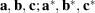Basis vectors of the crystal lattice; basis vectors of the reciprocal lattice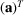Row of basis vectors [see Note (2)]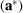Column of basis vectors of the reciprocal latticeX Point of point space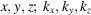Point coordinates; vector coefficients x; r Column of point coordinates; column of vector coefficients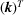Row of coefficients of a reciprocal-space vector [see Note (2)]Lengths of the basis vectors of the lattice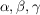Parameters of k-vector coefficients in CDML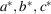Lengths of the basis vectors of the reciprocal lattice M, R, D, S Matrices W Matrix part of a mapping w Column part of a mapping (A, a), (W, w) Matrix–column pairs;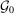;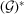Group or space group; symmorphic space group; reciprocal-space groupTranslation subgroup ofor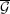;Point group; holohedral point groupSite-symmetry group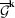;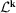Little co-group of k; little group of k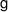,,Group elements of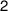,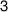,Symmetry operations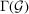(Matrix) representation of the groupNotes: (1) In crystallography, vectors are designated by lower-case bold-face letters. With K we make an exception in order to follow the tradition of physics. A crystallographic alternative could be. (2) In crystallography, point coordinates or vector coefficients are written as columns. Therefore, columns are taken as `normal'. In order to distinguish rows from columns (the coefficientsof vectors in reciprocal space, i.e. the Miller indices, and the basis of the crystal lattice are written as rows), rows are regarded as transposed columns and are thus marked by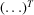.

References

International Tables for Crystallography (2005). Vol. A, Space-Group Symmetry, edited by Th. Hahn, 5th ed. Heidelberg: Springer.
Bradley, C. J. & Cracknell, A. P. (1972). The Mathematical Theory of Symmetry in Solids: Representation Theory for Point Groups and Space Groups. Oxford: Clarendon Press.
Cracknell, A. P., Davies, B. L., Miller, S. C. & Love, W. F. (1979). Kronecker Product Tables, Vol. 1, General Introduction and Tables of Irreducible Representations of Space Groups. New York: IFI/Plenum.